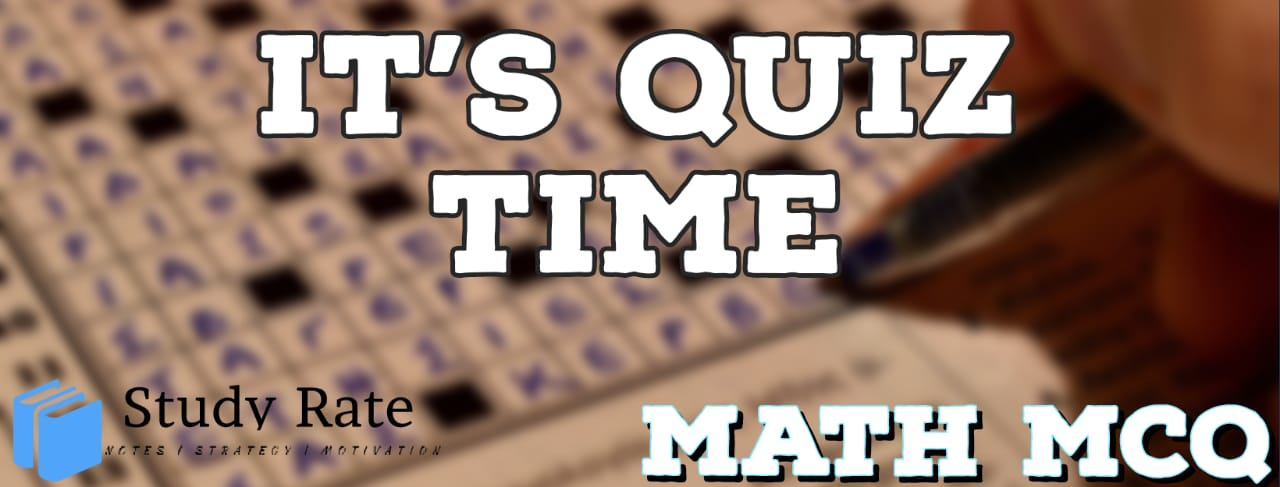## CBSE Class 10 Maths Chapter 11 – Constructions MCQ Quiz

Free PDF Download of CBSE Class 10 Maths Chapter 11 – Constructions Multiple Choice Questions with Answers. MCQ Questions for Class 10 Maths with Answers was Prepared Based on Latest Exam Pattern. Students can solve Class 10 Maths Constructions MCQs with Answers to know their preparation level

CBSE Board Class 10 Maths Chapter 11- Constructions Objective Questions

1. To divide a line segment AB in the ratio 5:7, first, a ray AX is drawn so that ∠BAX is an acute angle and then at equal distances points are marked on the ray AX such that the minimum number of these points is:

2. To divide a line segment AB in the ratio 4:7, a ray AX is drawn first such that ∠BAX is an acute angle and then points A1, A2, A3, …. are located at equal distances on the ray AX and the point B is joined to

3. To construct a triangle similar to a given ΔABC with its sides 8/5 of the corresponding sides of ΔABC draw a ray BX such that ∠CBX is an acute angle and X is on the opposite side of A with respect to BC. The minimum number of points to be located at equal distances on ray BX is:

4. To draw a pair of tangents to a circle which are inclined to each other at an angle of 60°, it is required to draw tangents at end points of those two radii of the circle, the angle between them should be:

5. To divide a line segment AB in the ratio p : q (p, q are positive integers), draw a ray AX so that ∠BAX is an acute angle and then mark points on ray AX at equal distances such that the minimum number of these points is

6. Match the following based on the construction of similar triangles, if scale factor (m/n) is

I. >1          a) The similar triangle is smaller than the original triangle.
II. <1        b) The two triangles are congruent triangles.
III. =1      c) The similar triangle is larger than the original triangle

7. The image of the construction of A’C’B a similar triangle of ΔACB is given below. Then choose the correct option:8. If a triangle similar to given ΔABC with sides equal to 3/4 of the sides of ΔABC is to be constructed, then the number of points to be marked on ray BX is __.9. Which of the following is not true for a point P on the circle?

10. There is a circle with center O. P is a point from where only one tangent can be drawn to this circle. What can we say about P?